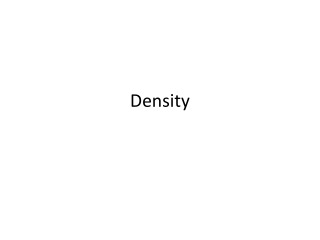DownloadDownload PresentationDensity

# Density

Télécharger la présentation## Density

- - - - - - - - - - - - - - - - - - - - - - - - - - - E N D - - - - - - - - - - - - - - - - - - - - - - - - - - -
##### Presentation Transcript

1. Density

2. - Amount of mass in a given volume grams Formula Calculate the density of an object that has a mass of 25.0 g and a volume of 4.7 mL. Given info: m = v = d = mL or cm3 g/mL or g/cm3 (3) 25.0 g 4.7 mL (2) ? g/mL 5.319 becomes 5.3 (2)

3. Calculate the mass of 20.0 cm3 of gold at a density of 19.3 g/cm3. Given info: m = ? g v = 20.0 cm3 d = 19.3 g/cm3 Do the algebra to solve for mass. m = vd m = (20.0 cm3)(19.3 g/cm3) m = 386 or 390 g with 2 sig figs

4. Determine the volume of air, in mL, in a balloon that has a mass of 6.73 g and a density of 1.32 g/L. Given info m = 6.73 g v = ? mL d = 1.32 g/L x Do the algebra to solve for volume. V = 5.10 x 103 mL The 5098.48 you get needs to be round off to show 3 sig figs. 5100 is only 2 sig figs therefore I wrote the answer in sci not. Must convert the L into mL. Remember since the L is on the bottom L has to go on top of the next step.